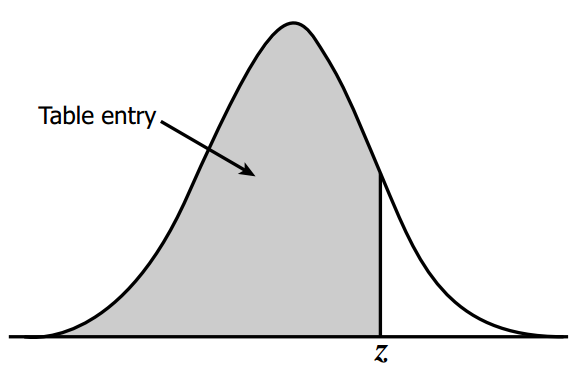Find z score

# HStatistics.com

### Find Z score

In this post we will look into how to find Z score from value of Z table.

Look at the image of Z table below.
The first column is the Z score value (till first decimal place) and the first row gives the second decimal place of the Z score.
All other values (from second row and second column onwards) are the percentage corresponding to the particular Z score.

For example, if I ask, what would be the Z score corresponding to 95% ?

So, you would look up the Z score corresponding to 95% in the Z table.
95% is basically 0.95 (95/100).

Look at the image of the Z table.
The highlighted blue box is the 95%. We need to find the Z score associated with this percentage.
Now look at the first column.

The Red box highlights the Z score (1.6 in this case) corresponding to 95%.
However, this Z score is only till the first decimal place. To find the second decimal place we need to look at the first row.
So the orange box highlighted in the first row gives us the second decimal place of the Z score corresponding to 95%.
Since 0.05 is highlighted in the orange box, we get total as 1.6 + 0.05 = 1.65 as the Z score corresponding to 95%.Another important point to note here is the meaning of this 95%.
This 95% means, we are finding the z-score at that point where the area covered by the graph (from the leftmost of the graph) is 95%.
As shown in the image below. The shaded area is what this percentage represents.This is how we find the Z score for a given percentage.
In the next post (here) , we will look into how to calculate this z score in different scenarios.